xPhase (waves)Encyclopedia
Phase in waves is the fraction of a wave cycle which has elapsed relative to an arbitrary point.

## Formula

The phase of an oscillation
Simple harmonic motion
Simple harmonic motion can serve as a mathematical model of a variety of motions, such as the oscillation of a spring. Additionally, other phenomena can be approximated by simple harmonic motion, including the motion of a simple pendulum and molecular vibration....

or wave
Sine wave
The sine wave or sinusoid is a mathematical function that describes a smooth repetitive oscillation. It occurs often in pure mathematics, as well as physics, signal processing, electrical engineering and many other fields...

refers to a sinusoidal function such as the following: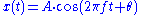where A, f, and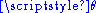are constant parameters. These functions are periodic with period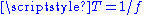, and they are identical except for a displacement of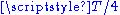along the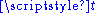axis. The term phase can refer to several different things:
• It can refer to a specified reference, such as, in which case we would say the phase of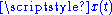is, and the phase of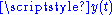is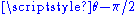.
• It can refer to, in which case we would sayandhave the same phase but are relative to different references.
• In the context of communication waveforms, the time-variant angle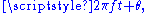or its modulovalue, is referred to as instantaneous phase
Instantaneous phase
The notions of Instantaneous Phase and Instantaneous Frequency are important concepts in Signal Processing that occur in the context of the representation and analysis of time-varying signals....

, but often just phase.  Instantaneous phase has a formal definition that is applicable to more general functions and unambiguously defines a function's initial phase at t=0.  Accordingly, it isforandfor.  (also see phasor)

## Phase shift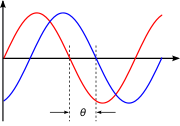Phase shift is any change that occurs in the phase of one quantity, or in the phase difference between two or more quantities.is sometimes referred to as a phase-shift, because it represents a "shift" from zero phase. But a change inis also referred to as a phase shift.

For infinitely long sinusoids, a change inis the same as a shift in time, such as a time-delay.  Ifis delayed (time-shifted) by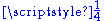of its cycle, it becomes: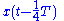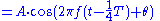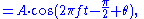whose "phase" is nowIt has been shifted byradians.

## Phase difference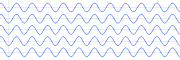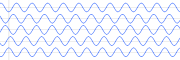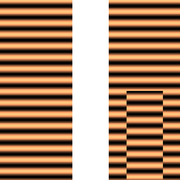Phase difference is the difference, expressed in electrical degrees or time, between two waves having the same frequency and referenced to the same point in time. Two oscillators that have the same frequency and different phases have a phase difference, and the oscillators are said to be out of phase with each other. The amount by which such oscillators are out of step with each other can be expressed in degree
Degree (angle)
A degree , usually denoted by ° , is a measurement of plane angle, representing 1⁄360 of a full rotation; one degree is equivalent to π/180 radians...

s from 0° to 360°, or in radian
Radian is the ratio between the length of an arc and its radius. The radian is the standard unit of angular measure, used in many areas of mathematics. The unit was formerly a SI supplementary unit, but this category was abolished in 1995 and the radian is now considered a SI derived unit...

s from 0 to 2π. If the phase difference is 180 degrees (π radians), then the two oscillators are said to be in antiphase. If two interacting wave
Wave
In physics, a wave is a disturbance that travels through space and time, accompanied by the transfer of energy.Waves travel and the wave motion transfers energy from one point to another, often with no permanent displacement of the particles of the medium—that is, with little or no associated mass...

s meet at a point where they are in antiphase, then destructive interference will occur. It is common for waves of electromagnetic (light, RF), acoustic (sound) or other energy to become superposed in their transmission medium. When that happens, the phase difference determines whether they reinforce or weaken each other. Complete cancellation is possible for waves with equal amplitudes.

Time is sometimes used (instead of angle) to express position within the cycle of an oscillation.
• A phase difference is analogous to two athletes running around a race track at the same speed and direction but starting at different positions on the track. They pass a point at different instants in time. But the time difference (phase difference) between them is a constant - same for every pass since they are at the same speed and in the same direction. If they were at different speeds (different frequencies), the phase difference is undefined and would only reflect different starting positions. Technically, phase difference between two entities at various frequencies is undefined and does not exist.
• Time zones are also analogous to phase differences.

## In-phase and quadrature (I&Q) components

The term in-phase is also found in the context of communication signals: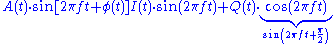and: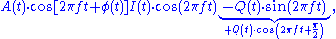whererepresents a carrier frequency
Carrier wave
In telecommunications, a carrier wave or carrier is a waveform that is modulated with an input signal for the purpose of conveying information. This carrier wave is usually a much higher frequency than the input signal...

, and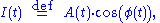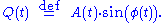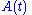and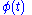represent possible modulation
Modulation
In electronics and telecommunications, modulation is the process of varying one or more properties of a high-frequency periodic waveform, called the carrier signal, with a modulating signal which typically contains information to be transmitted...

of a pure carrier wave, e.g.: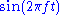(or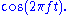) The modulation alters the original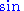(or) component of the carrier, and creates a (new)(or) component, as shown above. The component that is in phase with the original carrier is referred to as the in-phase component. The other component, which is always 90° (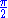radians) "out of phase", is referred to as the quadrature component.

## Phase coherence

Coherence
Coherence (physics)
In physics, coherence is a property of waves that enables stationary interference. More generally, coherence describes all properties of the correlation between physical quantities of a wave....

is the quality of a wave to display well defined phase relationship in different regions of its domain of definition.

In physics, quantum mechanics
Quantum mechanics
Quantum mechanics, also known as quantum physics or quantum theory, is a branch of physics providing a mathematical description of much of the dual particle-like and wave-like behavior and interactions of energy and matter. It departs from classical mechanics primarily at the atomic and subatomic...

ascribes waves to physical objects. The wave function is complex and since its square modulus is associated with the probability of observing the object, the complex character of the wave function is associated to the phase. Since the complex algebra is responsible for the striking interference effect of quantum mechanics, phase of particles is therefore ultimately related to their quantum behavior.

## Phase compensation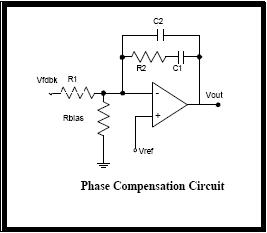Phase compensation is the correction of phase error (i.e., the difference between the actually needed phase and the obtained phase). A phase compensation is required to obtain stability in an opamp. A capacitor/RC network is usually used in the phase compensation to keep a phase margin. A phase compensator subtracts out an amount of phase shift from a signal which is equal to the amount of phase shift added by switching one or more additional amplifier stages into the amplification signal path.

• Instantaneous phase
Instantaneous phase
The notions of Instantaneous Phase and Instantaneous Frequency are important concepts in Signal Processing that occur in the context of the representation and analysis of time-varying signals....

• Lissajous curve
Lissajous curve
In mathematics, a Lissajous curve , also known as Lissajous figure or Bowditch curve, is the graph of a system of parametric equationswhich describe complex harmonic motion...

• Phase angle
Phase angle
In the context of vectors and phasors, the term phase angle refers to the angular component of the polar coordinate representation. The notation A\ang \!\ \theta,   for a vector with magnitude A and phase angle θ, is called angle notation.In the context of periodic phenomena, such as a wave,...

• Phase cancellation
• Phase velocity
Phase velocity
The phase velocity of a wave is the rate at which the phase of the wave propagates in space. This is the speed at which the phase of any one frequency component of the wave travels. For such a component, any given phase of the wave will appear to travel at the phase velocity...

• Polarity
• Polarization